• 判断是否为数字
2015-08-04 13:52:57

c++检测输入的是否为数字：

代码：

bool isNum(const string & str)
{	const char *ch = str.c_str();
if (*ch <='Z' && *ch >= 'A' || *ch <='z' && *ch >='a')
{
return false;
}
else if(*ch >='0' && *ch <='9')
{
return true;
}
}C++ c语言
更多相关内容
• Matlab GUI 界面编程：判断可编辑文本框输入的是否为数字 20191206 在可编辑文本框的回调函数中： input = str2num(get(handles.edit1,'string')); if isempty(input) msgbox('请输入数字','确认','error'); set...

### Matlab GUI 界面编程：判断可编辑文本框输入的是否为数字

20191206

在可编辑文本框的回调函数中：

input = str2num(get(handles.edit1,'string'));
if isempty(input)
msgbox('请输入数字','确认','error');
set(handles.edit1,'string',''); % 将内容清空
end

展开全文• ## MATLAB判断字符类型

千次阅读 2021-02-06 10:32:07
文章目录一、判断是否为字母类型二、判断是否为空格字符三、判断多种字符类型四、字符串转数字例题 一、判断是否为字母类型   isletter（）函数：‘1’表示字符串对应的位置字母字符，‘0’则相反 如何提取...

# 一、判断是否为字母类型

isletter（）函数：‘1’表示字符串对应的位置为字母字符，‘0’则相反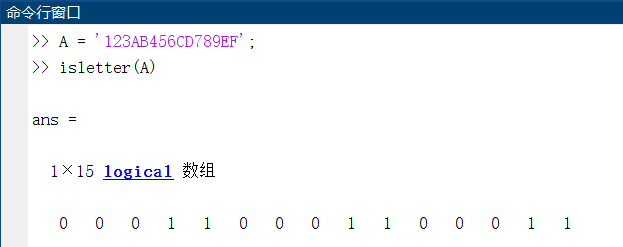如何提取字符串当中全部的英文字符呢？解决方法如下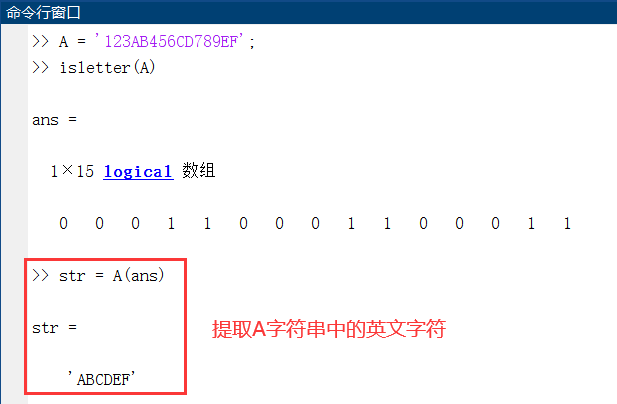那想要提取字符串中 i 到 j 位置的字母字符时又该怎么办呢？
代码如下：

A = '123AB456CD789EF';
M = isletter(A);
str = '';						#提前申明空字符串str
for i = 5:13					#假设i==5,j==13
if M(i) == 1
str = [str A(i)];		#将str字串与A(i)字符拼接成新的字符串
end
end
str								#查看最终的string


实现结果如下：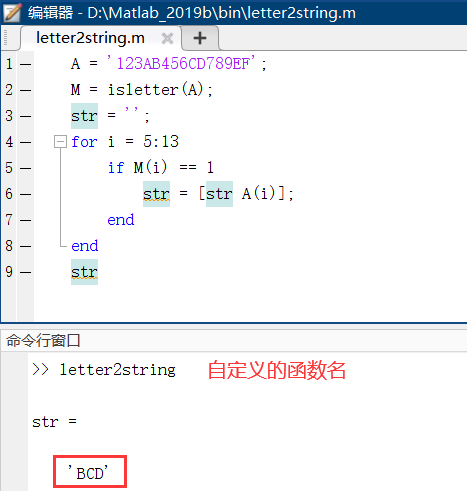# 二、判断是否为空格字符

isspace（）函数：‘1’表示字符串对应的位置为空格字符，‘0’则相反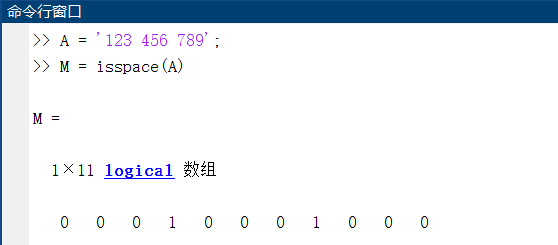那么老问题来了，如果你想去除字符串当中的空格字符怎么办呢？

代码如下（示例）：

A = '123 456 789';
M = isspace(A);					#判断空格字符
str = '';						#申明初始字符串
for i = 1:length(A)				#length(A)为A字符串的长度
if M(i) ~= 1				#M(i)非1，代表字符串该位置不是空格字符
str = [str A(i)];		#进行字符串拼接
end
end
str								#查看最终字符串


实现结果如下：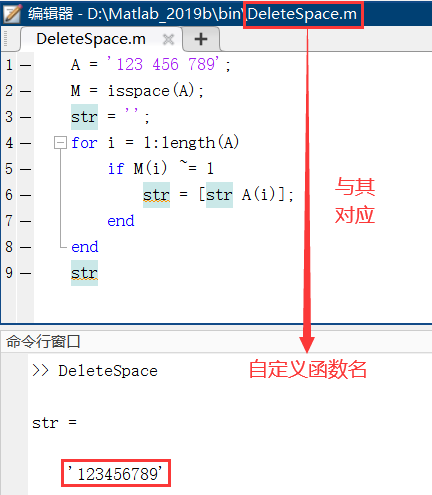# 三、判断多种字符类型

isstrprop（）函数：‘1’表示字符串对应的位置为相应字符，‘0’则相反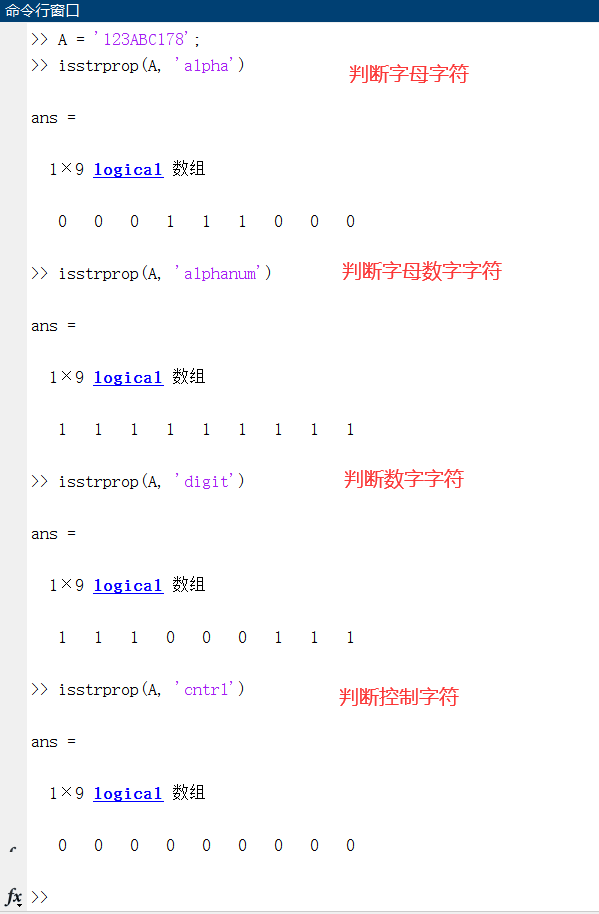# 四、字符串转数字例题

华氏温度转摄氏温度
输入字符串，如果字符串是数字字符串，那么就进行温度转换
如果字符串不是数字字符串，那么就输出 ‘输入有误，请输入数字’
如果字符串是空字符串，那么结束程序

代码示例如下：

function F2C()
while 1
str = input("Temperature in F: ",'s');
if isempty(str)
disp('程序结束');
break;
else
if isnan(str2double(str))
disp('输入有误，请输入数字');
continue;
else
temperature = (str2double(str) - 32)*5/9;
disp(['------>Temperature in C = ',num2str(temperature,'%.2f'),'°C']);
end
end
end


实现结果如下：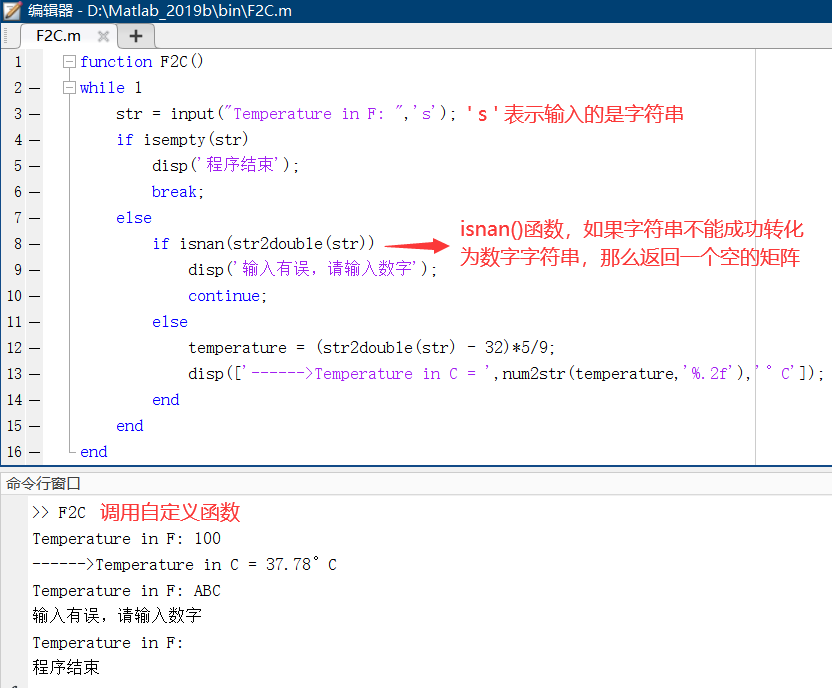展开全文矩阵 字符串
• 自己选用的是第一个 原始参考链接

自己选用的是第一个原始参考链接

展开全文• isstr是判断string isnumeric是判断number number与string之间的转换 http://cn.mathworks.com/help/matlab/ref/str2num.html http://cn.mathworks.com/help/matlab/ref/str2num.html cellfun函数的应用 ...
• Membership三步曲之入门篇 - ...发送http请求 如何拼接想要的url http://weixin.sogou.com/weixin?type=1&page=1&ie=utf8&query=%E5%A4%A7%E7%BA%BD ... Go 实现判断变量是否为合法数字 IsNumeric 算法 [转] ...
• 否则逻辑 0 (FALSE)。 如果定义了 A 并且您设置了 B = A，则 STRUCTCMP(A,B) 不一定是真的。 如果 A 或 B 的字段包含 NaN（非数字）元素，则 STRUCTCMP 返回false 因为 NaN 根据定义彼此不相等。 认为 NaN 值相等...
• all(A==0) 如果向量A全为0，返回1，否则返回0
• MATLAB中，定义一个变量非数值类型的语句： a = NaN; 在判断变量是否为非数值类型时，不能通过 ‘==’ 符号来判断，需要通过isnan函数进行判断，格式： isnan(a)
• if(x == floor(x) && x == ceil(x)) end 这样做的好处是对包括0在内的正负整数均适用
• MATLAB 判断元素是否在矩阵中 matlab里面真是好多操作都不支持，没有python做处理那么灵活 判断元素还是有函数的——ismember b = ismember(a,A) %% 以下是在命令行下操作 >> A = [1,2,3;4,5,6] A = 1 2 3 ...
• ## matlab判断语句与

千次阅读 2021-04-27 01:28:42
matlab if语句在MATLAB中，if语句有3种格式。(1) 单分支if语句：if 条件 语句组 end当条件成立时，则执行语句组，执行完之后继续执行if语句的后继语句，若条件不成立，则直接执行if语句的后继语句。 (2) 双分支if...
• 假设变量a，使用以下语句进行判断： 参考链接：MATLAB判断一个矩阵或者数字是否是复数 if ~isreal(a)
• 任务描述：我有一个站点观测降水的时间序列，需要判断降水观测是否连续出现10天以上的缺省值 写成了函数，直接调用即可 %% 本函数用于判断某数连续出现的次数超过某次， function result = default_test(A,B,count) ...
• 需要在输入之前，加一个判断，查看图像的通道数。 之前是拿来主义，不懂什么这样写，现在懂了，写一个笔记。 clear;close all;clc; I = imread('color.jpg'); img_size = size(I) % 想记录的其实就这两行：先要...计算机视觉 图像处理
• matlab中如何判断两个数据的值是否相等呢？我们都知道通常使用isequal()方法，调用方法如下： tf = isequal(A, B) 然而数据在什么情况下是相等的呢？我们看如下情况 x = 1.38389652673674e-20; y = 1....
• 但是我的目的是，比如10,11,12记4，那我想知道当序号4时，对应原来数组中的序号10,11,12中最小的是哪个，即得到10。如果您有时间能不能帮我解释修改下呢? 再答： l=length(a); m=1; aa=num2str(a(1)); for k=2:l...
• 测试八位数字是否为有效日期 String : Regex : [Ctrl+A 全选 注:如需引入外部Js需刷新才能执行]正则
• 对于带有数字列表的两个向量，找到重复的实体。 例子： [aNew,bNew] = findDuplicate([1.2 2.4 6.3 6.2],[1.2 1.2 6.2 5])
• matlab : R2018a 64bitOS : Windows 10 x64typesetting : Markdownblog : my.oschina.net/zhichengjiu判断子集codeclearclc% 判断a是不是b的子集a=[1 2 3 4 5];b=[1 2 3 4 5 6];c=ismember(a,b)d=all(c);if(d==1)...
• 输入可能空，字符型，数字型，字符型有肯能无法转换成数字。针对以上情况，编制一个子程序进行判断，输出结果。 function [output, error_code] = input2num(input) % 程序功能： 对输入进行判断，给出对应的错误...
• 判断Python输入是否为数字 在接收raw_input方法后，判断接收到的字符串是否为数字 例如： str = raw_input(“please input the number:”) if str.isdigit(): True表示输入的所有字符都是数字，否则，不是全部...python 开发语言
• isOdd 确定一个数字是奇数还是偶数。 isOdd 接受一个数字数组，并返回一个相同的逻辑数组size 作为输入，其中真 (1) 是奇数，假 (0) 是偶数。 tf = isOdd(5) tf = 1 tf = isOdd([1 2 3]) tf = 1 0 1 由大卫·考文垂...
• 这个脚本找到了简单明了的快乐数字，虽然范围大于 100000，但可以使用一些优化。
• 判断方法：X=sum(sum(Z)),所得的X值即负数的个数。 原理解释： 1.Z表示将Z中的各个元素与0相比较，若元素小于0则返回1，否则若元素大于0则返回0。 代码实现： 结果显示： 2.sum(Z):表示将Z这一个判断矩阵按列求和...矩阵
• 使用matlab 判断两个矩阵是否相等的实例数学意义的相等all(A(:) == B(:))isequal(A, B)但须注意的是：B = A，未必能保证 isequal(A, B)返回真，因为如果 A 中包含NaN，因为按照定义，NaN ~= NaN>> A = [1, NaN...
• matlab程序 判断一个数是否为质数 参数以及详细描述都在代码里了
• all函数：检测矩阵中是否全为非零元素，如果是，则返回1，否则，返回0。 any函数：检测矩阵中是否有非零元素，如果有，则返回1，否则，返回0。...

# matlab判断是否为数字matlab 订阅